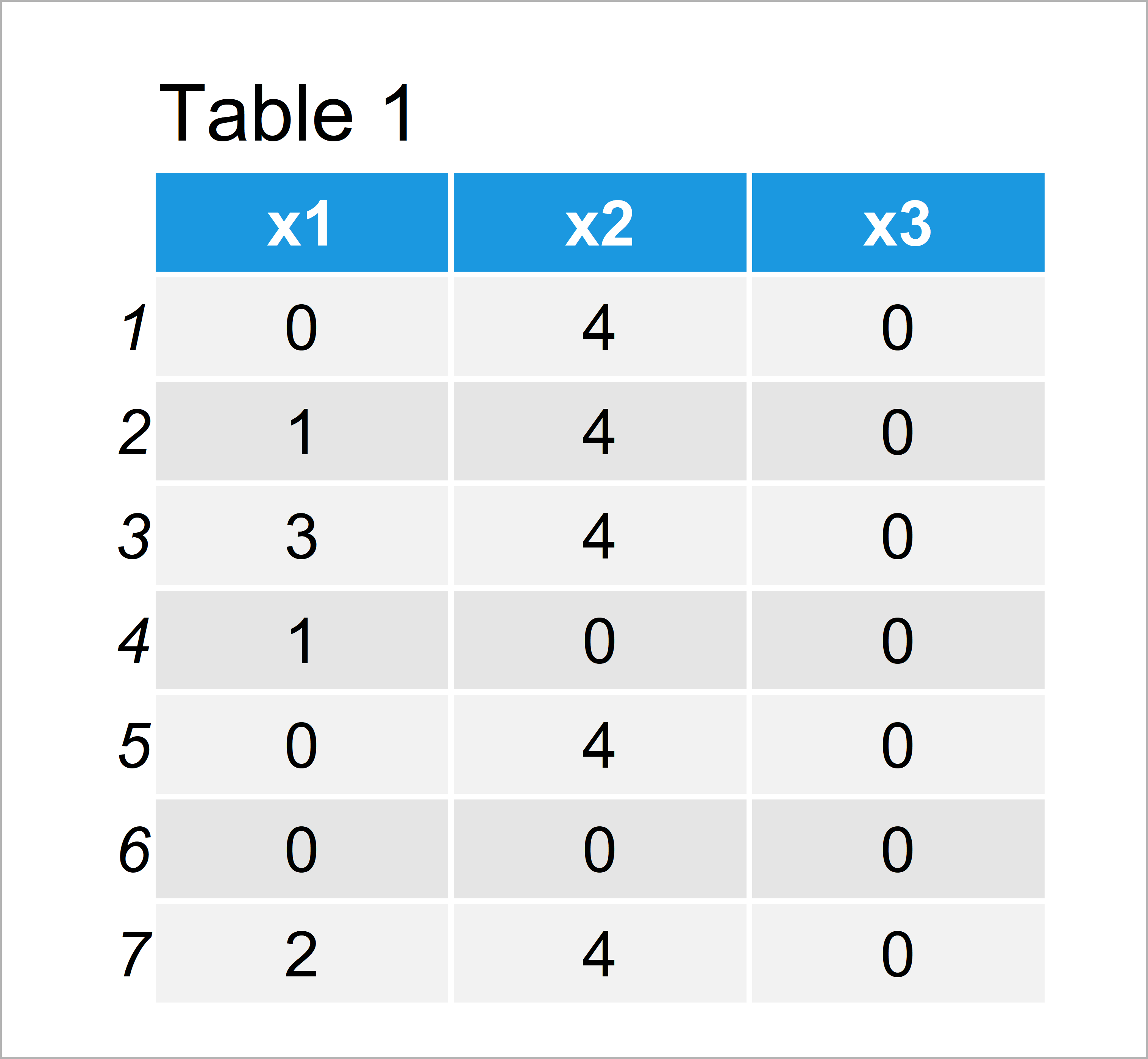# Count Non-Zero Values in Vector & Data Frame Columns in R (2 Examples)

In this tutorial, I’ll show how to get the number of non-zero values in the R programming language.

Here’s the step-by-step process:

## Example 1: Count Non-Zero Values in Vector Object

This example shows how to return the number of non-zero values in a vector.

Consider the following example vector in R:

```vec <- c(3, 0, 0, 4, 1, 0, 2, 0, 3)                # Create example vector
vec                                                # Print example vector
#  3 0 0 4 1 0 2 0 3```

If we now want to count the number of values unequal to zero, we can use the != operator and the sum function as shown below:

```sum(vec != 0)                                      # Count non-zeros
#  5```

The RStudio console returns the value 5, i.e. five elements of our vector are unequal to zero.

## Example 2: Count Non-Zero Values in Each Data Frame Column

In Example 2, I’ll explain how to count the number of non-zero values in each column of a data frame.

Let’s create some example data:

```data <- data.frame(x1 = c(0, 1, 3, 1, 0, 0, 2),    # Create example data frame
x2 = c(4, 4, 4, 0, 4, 0, 4),
x3 = 0)
data                                               # Print example data frame```The output of the previous syntax is shown in Table 1: We have created a data frame with seven rows and three columns.

Next, we can apply the != operator in combination with the colSums function to return the number of non-zero values in each variable of our data frame:

```colSums(data != 0)                                 # Count non-zeros
# x1 x2 x3
#  4  5  0```

The column x1 contains four non-zero values, the column x2 contains five values that are not equal to zero, and the column x3 contains only zeros.

## Video, Further Resources & Summary

If you need further information on the R programming syntax of this article, I recommend having a look at the following video that I have published on my YouTube channel. I illustrate the contents of this article in the video.

Please accept YouTube cookies to play this video. By accepting you will be accessing content from YouTube, a service provided by an external third party.If you accept this notice, your choice will be saved and the page will refresh.

Also, you might read the related articles on my website. A selection of articles can be found below.

In this tutorial you have learned how to count non-zeros in vectors and data frame columns in the R programming language. Tell me about it in the comments, in case you have any additional questions.

Subscribe to the Statistics Globe Newsletter